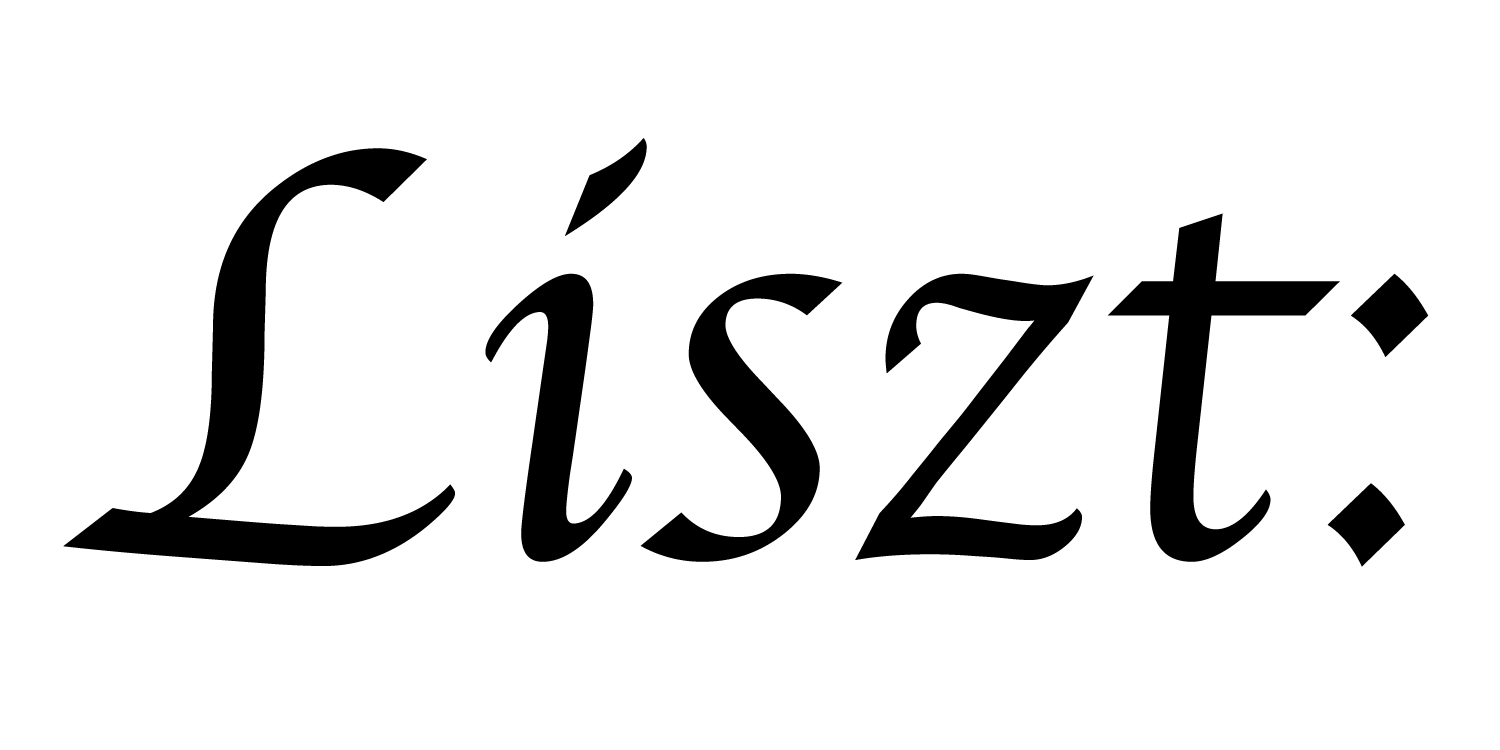# 17: Subsets

In tutorial 07, computing heat diffusion using the standard grid library, we imposed boundary conditions instead of having both directions be periodic. To do so, we made use of subsets. In this tutorial, we’ll see how to define and use subsets of a relation.

``````import 'ebb'
local L = require 'ebblib'

local vdb   = require('ebb.lib.vdb')

local N = 50

local cells = L.NewRelation {
name      = 'cells',
dims      = { N, N },
periodic  = { false, false },
}

cells:NewFieldMacro('__apply_macro', L.Macro(function(c, x, y)
return ebb `L.Affine(cells, { {1,0, x},
{0,1, y} }, c)
end))
``````

We’ll start the program by creating a cells relation without any periodicity, and define an offset macro.

``````local timestep    = L.Constant(L.double, 0.04)
local conduction  = L.Constant(L.double, 1.0)
local max_diff    = L.Global(L.double, 0.0)

if xi == 4 and yi == 10 then return 1000 else return 0 end
end)

local ebb compute_step( c : cells )
var avg_t = ( c(1,0).t + c(-1,0).t
+ c(0,1).t + c(0,-1).t ) / 4.0
var d_t   = avg_t - c.t
c.t_next  = c.t + timestep * conduction * d_t
end
``````

Here we define the basic simulation variables, fields, and core update function.

However, the update function has a problem. If we simply execute `cells:foreach(compute_step)` then all of the cells on the boundary will try to access neighbors that don’t exist, resulting in the equivalent of array-out-of-bounds errors. These might manifest as segmentation faults, bad data, or in any number of other ways.

``````cells:NewSubset('interior', { {1,N-2}, {1,N-2} })
``````

Instead of executing `compute_step()` over all the cells, we want to execute it only over the “interior” cells. Ebb lets us define this subset using the `NewSubset()` function. We pass the function a name for the subset, and a list of (inclusive) ranges specifying a rectangular subset of the grid.

``````cells:NewSubset('boundary', {
rectangles = { { {0,0},     {0,N-1}   },
{ {N-1,N-1}, {0,N-1}   },
{ {0,N-1},   {0,0}     },
{ {0,N-1},   {N-1,N-1} } }
})
``````

Instead of defining a subset by specifying a single rectangle, we can also specify a set of rectangles. Here we use four rectangles to specify the left, right, bottom and top boundaries of the grid. This is the complement of the ‘interior’ subset.

``````cells:NewFieldReadFunction('is_left_bd',   ebb (c) return L.xid(c) == 0 end)
cells:NewFieldReadFunction('is_right_bd',  ebb (c) return L.xid(c) == N-1 end)
cells:NewFieldReadFunction('is_bottom_bd', ebb (c) return L.yid(c) == 0 end)
cells:NewFieldReadFunction('is_top_bd',    ebb (c) return L.yid(c) == N-1 end)
``````

Within the boundary, we want to be able to identify which side(s) a cell is on. We hide these tests behind field functions so that the meaning of the code is more clear.

``````local ebb compute_neumann_boundary_update( c : cells )
var sum_t = 0.0
if not c.is_left_bd   then sum_t += c(-1,0).t
else sum_t += c.t end
if not c.is_right_bd  then sum_t += c(1,0).t
else sum_t += c.t end
if not c.is_bottom_bd then sum_t += c(0,-1).t
else sum_t += c.t end
if not c.is_top_bd    then sum_t += c(0,1).t
else sum_t += c.t end
var d_t = sum_t / 4.0 - c.t
c.t_next  = c.t + timestep * conduction * d_t
end
``````

A Neumann boundary condition specifies a zero-derivative at the boundary in the direction of the boundary. That is, the flux is 0, or put another way, no heat should leave or enter the simulation. (We can test this.) We simulate this condition by having non-existant neighbors assume the same temperature value as the centered cell. (i.e. a difference of 0)

Notice that if we execute this function over all of the cells, we will compute the same result for interior cells as the `compute_step()` function. Depending on a variety of factors in the implementation and hardware, this may be a more or less efficient approach. (You can test the difference below) If these branches contain much more math and we run on a GPU, then launching over seperate subsets is likely to be much more efficient.

``````local max_diff = L.Global(L.double, 0.0)
local sum_t    = L.Global(L.double, 0.0)

local ebb measure_diff ( c : cells )
var diff = L.fmax( L.fmax( L.fabs(c.t - c(0,0).t),
L.fabs(c.t - c(0,1).t) ),
L.fmax( L.fabs(c.t - c(1,0).t),
L.fabs(c.t - c(1,1).t) ))
max_diff max= diff
end
local ebb measure_sum ( c : cells )
sum_t += c.t
end

local ebb visualize ( c : cells )
vdb.color({ 0.5 * c.t + 0.5, 0.5-c.t, 0.5-c.t })
vdb.point({ L.xid(c), L.yid(c), 0 })
end
``````

visualization and statistics functions are defined above.

``````for i=1,20000 do
cells.interior:foreach(compute_step)
cells.boundary:foreach(compute_neumann_boundary_update)
--cells:foreach(compute_neumann_boundary_update)
cells:Swap('t','t_next')

if i % 1000 == 0 then -- measure statistics and visualize every 1000 steps
vdb.vbegin()
vdb.frame()
cells:foreach(visualize)
vdb.vend()

max_diff:set(0)
sum_t:set(0)
cells:foreach(measure_sum)
cells:foreach(measure_diff)
print( 'iteration #'..tostring(i),